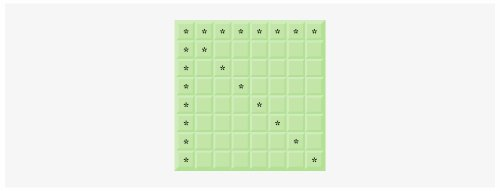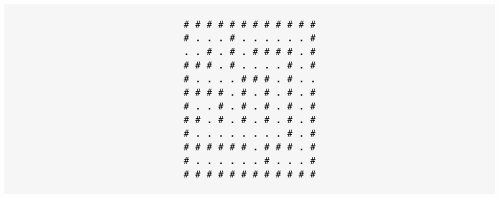# Internet and Web Resources

### Answers to Self Review Exercises

 15.1 a) False. A method that calls itself indirectly is an example of recursionmore specifically, an example of indirect recursion. b) False. Recursion can be inefficient in computation because of multiple method calls and memory space usage. c) True. d) False. To make recursion feasible, the recursion step in a recursive solution must resemble the original problem, but be a slightly smaller version of it. 15.2 d 15.3 a 15.4 c 15.5 b 15.6 a) golden ratio, golden mean. b) top. c) last-in, first-out (LIFO). d) activation record, stack frame. e) stack overflow. f) selection. g) self-similar. h) permutations. i) method-call.

### Exercises

 15.7 What does the following code do? ``` 1 public int mystery( int a, int b ) 2 { 3 if ( b == 1 ) 4 return a; 5 else 6 return a + mystery( a, b - 1 ); 7 } // end method mystery ``` 15.8 Find the error(s) in the following recursive method, and explain how to correct it (them). This method should find the sum of the values from 0 to n. ``` 1 public int sum( int n ) 2 { 3 if ( n == 0 ) 4 return 0; 5 else 6 return n + sum( n ); 7 } // end method sum ``` 15.9 (Recursive power Method) Write a recursive method power( base, exponent ) that, when called, returns baseexponent For example, power( 3,4 ) = 3 * 3 * 3 * 3. Assume that exponent is an integer greater than or equal to 1. [Hint: The recursion step should use the relationship baseexponent = base · baseexponent 1 and the terminating condition occurs when exponent is equal to 1, because base1 = base Incorporate this method into a program that enables the user to enter the base and exponent.] 15.10 (Visualizing Recursion) It is interesting to watch recursion "in action." Modify the factorial method in Fig. 15.3 to print its local variable and recursive-call parameter. For each recursive call, display the outputs on a separate line, and add a level of indentation. Do your utmost to make the outputs clear, interesting and meaningful. Your goal here is to design and implement an output format that makes it easier to understand recursion. You may want to add such display capabilities to other recursion examples and exercises throughout the text. 15.11 (Greatest Common Divisor) The greatest common divisor of integers x and y is the largest integer that evenly divides into both x and y. Write a recursive method gcd that returns the greatest common divisor of x and y. The gcd of x and y is defined recursively as follows: If y is equal to 0, then gcd( x, y ) is x; otherwise, gcd( x, y ) is gcd( y, x % y ), where % is the remainder operator. Use this method to replace the one you wrote in the application of Exercise 6.27. 15.12 What does the following program do? ``` 1 // Exercise 15.12 Solution: MysteryClass.java 2 3 public class MysteryClass 4 { 5 public int mystery( int array2[], int size ) 6 { 7 if ( size == 1 ) 8 return array2[ 0 ]; 9 else 10 return array2[ size - 1 ] + mystery( array2, size - 1 ); 11 } // end method mystery 12 } // end class MysteryClass ```   ``` 1 // Exercise 15.12 Solution: MysteryTest.java 2 3 public class MysteryTest 4 { 5 public static void main( String args[] ) 6 { 7 MysteryClass mysteryObject = new MysteryClass(); 8 9 int array[] = { 1, 2, 3, 4, 5, 6, 7, 8, 9, 10 }; 10 11 int result = mysteryObject.mystery( array, array.length ); 12 13 System.out.printf( "Result is: %d ", result ); 14 } // end method main 15 } // end class MysteryTest ``` 15.13 What does the following program do? ``` 1 // Exercise 15.13 Solution: SomeClass.java 2 3 public class SomeClass 4 { 5 public String someMethod( 6 int array2[], int x, String output ) 7 { 8 if ( x < array2.length ) 9 return String.format( 10 "%s%d ", someMethod( array2, x + 1 ), array2[ x ] ); 11 else 12 return ""; 13 } // end method someMethod 14 } // end class SomeClass ```   ``` 1 // Exercise 15.13 Solution: SomeClassTest.java 2 3 public class SomeClassTest 4 { 5 public static void main( String args[] ) 6 { 7 SomeClass someClassObject = new SomeClass(); 8 9 int array[] = { 1, 2, 3, 4, 5, 6, 7, 8, 9, 10 }; 10 11 String results = 12 someClassObject.someMethod( array, 0 ); 13 14 System.out.println( results ); 15 } // end main 16 } // end class SomeClassTest ``` 15.14 (Palindromes) A palindrome is a string that is spelled the same way forwards and backwards. Some examples of palindromes are "radar," "able was i ere i saw elba" and (if spaces are ignored) "a man a plan a canal panama." Write a recursive method testPalindrome that returns boolean value true if the string stored in the array is a palindrome and false otherwise. The method should ignore spaces and punctuation in the string. 15.15 (Eight Queens) A puzzler for chess buffs is the Eight Queens problem, which asks the following: Is it possible to place eight queens on an empty chessboard so that no queen is "attacking" any other (i.e., no two queens are in the same row, in the same column or along the same diagonal)? For instance, if a queen is placed in the upper-left corner of the board, no other queens could be placed in any of the marked squares shown in Fig. 15.25. Solve the problem recursively. [Hint: Your solution should begin with the first column and look for a location in that column where a queen can be placedinitially, place the queen in the first row. The solution should then recursively search the remaining columns. In the first few columns, there will be several locations where a queen may be placed. Take the first available location. If a column is reached with no possible location for a queen, the program should return to the previous column, and move the queen in that column to a new row. This continuous backing up and trying new alternatives is an example of recursive backtracking.] Figure 15.25. Squares eliminated by placing a queen in the upper-left corner of a chessboard.15.16 (Print an Array) Write a recursive method printArray that displays all the elements in an array of integers, separated by spaces. 15.17 (Print an Array Backwards) Write a recursive method stringReverse that takes a character array containing a string as an argument and prints the string backwards. [Hint: Use String method toCharArray, which takes no arguments, to get a char array containing the characters in the String.] 15.18 (Find the Minimum Value in an Array) Write a recursive method recursiveMinimum that determines the smallest element in an array of integers. The method should return when it receives an array of one element. 15.19 (Fractals) Repeat the fractal pattern in Section 15.9 to form a star. Begin with five lines, instead of one, where each line is a different arm of the star. Apply the "Lo fractal" pattern to each arm of the star. 15.20 (Maze Traversal Using Recursive Backtracking) The grid of #s and dots (.) in Fig. 15.26 is a two-dimensional array representation of a maze. The #s represent the walls of the maze, and the dots represent locations in the possible paths through the maze. Moves can be made only to a location in the array that contains a dot. Figure 15.26. Two-dimensional array representation of a maze.Write a recursive method (mazeTraversal) to walk through mazes like the one in Fig. 15.26. The method should receive as arguments a 12-by-12 character array representing the maze and the current location in the maze (the first time this method is called, the current location should be the entry point of the maze). As mazeTraversal attempts to locate the exit, it should place the character x in each square in the path. There is a simple algorithm for walking through a maze that guarantees finding the exit (assuming there is an exit). If there is no exit, you will arrive at the starting location again. The algorithm for this method is as follows: From the current location in the maze, try to move one space in any of the possible directions (down, right, up or left). If it is possible to move in at least one direction, call mazeTraversal recursively, passing the new spot on the maze as the current spot. If it is not possible to go in any directions, "back up" to a previous location in the maze and try a new direction for that location. Program the method to display the maze after each move so the user can watch as the maze is solved. The final output of the maze should display only the path needed to solve the mazeif going in a particular direction results in a dead end, the x's going in that direction should not be displayed. [Hint: To display only the final path, it may be helpful to mark off spots that result in a dead end with another character (such as '0').] 15.21 (Generating Mazes Randomly) Write a method mazeGenerator that takes as an argument a two-dimensional 12-by-12 character array and randomly produces a maze. The method should also provide the starting and ending locations of the maze. Test your method mazeTraversal from Exercise 15.20, using several randomly generated mazes. 15.22 (Mazes of Any Size) Generalize methods mazeTraversal and mazeGenerator of Exercise 15.20 and Exercise 15.21 to process mazes of any width and height. 15.23 (Time to Calculate Fibonacci Numbers) Enhance the Fibonacci program of Fig. 15.5 so that it calculates the approximate amount of time required to perform the calculation and the number of calls made to the recursive method. For this purpose, call static System method currentTimeMillis, which takes no arguments and returns the computer's current time in milliseconds. Call this method twiceonce before the call to fibonacci and once after the call to fibonacci. Save each of these values and calculate the difference in the times to determine how many milliseconds were required to perform the calculation. Then, add a variable to the FibonacciCalculator class, and use this variable to determine the number of calls made to method fibonacci. Display your results.Java How to Program (6th Edition) (How to Program (Deitel))
ISBN: 0131483986
EAN: 2147483647
Year: 2003
Pages: 615